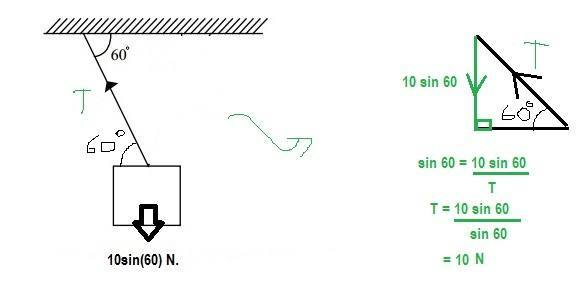# Tension of rope

haruspex
Homework Helper
Gold Member
2020 Award
View attachment 91592

the ans is 10N after cut the 5N right rope...huhu near but still cannot get the ans
After a rope is cut, the system is no longer stable. There will be an acceleration. The forces in the vertical direction will no longer balance.
You need to consider which way that acceleration will be and apply ##\Sigma F=0## at right angles to it.

After a rope is cut, the system is no longer stable. There will be an acceleration. The forces in the vertical direction will no longer balance.
You need to consider which way that acceleration will be and apply ##\Sigma F=0## at right angles to it.
if one of the ropes have been cut, it mean the object just hang VERTICALLY with one rope with 10sin(60)N load on it.Is it my assumption is correct?

it mean all the calculation in step 25 not require especially step 2 &3 ???

haruspex
Homework Helper
Gold Member
2020 Award
if one of the ropes have been cut, it mean the object just hang VERTICALLY with one rope with 10sin(60)N load on it.Is it my assumption is correct?
Not immediately. Although the question does not make it completely clear, I am very confident that you are supposed to find the tension in the remaining rope the instant after one rope is cut. You can take the remaining rope and the mass as being in exactly the same positions as before, and, for the instant, stationary. But now there is a nonzero acceleration.
Which way will the mass accelerate?

•stuc
Not immediately. Although the question does not make it completely clear, I am very confident that you are supposed to find the tension in the remaining rope the instant after one rope is cut. You can take the remaining rope and the mass as being in exactly the same positions as before, and, for the instant, stationary. But now there is a nonzero acceleration.
Which way will the mass accelerate?

Do you mean the answer is wrong (Given ans: 5N)..i still stuck

haruspex
Homework Helper
Gold Member
2020 Award
Do you mean the answer is wrong (Given ans: 5N)..i still stuck
Yes, it's wrong. (That would be the right answer if the angle were 45 degrees.)
You have established that the weight is 10sin(60) N. Draw a free body diagram, exactly like the original diagram, but with only one rope.
So the rope is still at 60 degrees to the horizontal.
Let the tension in the rope be T. You know the weight, and hence the mass. The tension and the weight are the only forces acting, agreed?
Which way will the mass accelerate?
What can you therefore say about the forces orthogonal to that direction?

•stuc
Yes, it's wrong. (That would be the right answer if the angle were 45 degrees.)
You have established that the weight is 10sin(60) N. Draw a free body diagram, exactly like the original diagram, but with only one rope.
So the rope is still at 60 degrees to the horizontal.
Let the tension in the rope be T. You know the weight, and hence the mass. The tension and the weight are the only forces acting, agreed?
Which way will the mass accelerate?
What can you therefore say about the forces orthogonal to that direction?

thank you very much for your guide..i will try your idea. i hope you have time to check it

thank you very much for your guide..i will try your idea. i hope you have time to check it
Yes, it's wrong. (That would be the right answer if the angle were 45 degrees.)
You have established that the weight is 10sin(60) N. Draw a free body diagram, exactly like the original diagram, but with only one rope.
So the rope is still at 60 degrees to the horizontal.
Let the tension in the rope be T. You know the weight, and hence the mass. The tension and the weight are the only forces acting, agreed?
Which way will the mass accelerate?
What can you therefore say about the forces orthogonal to that direction?dear haruspex ... is it like this???

haruspex